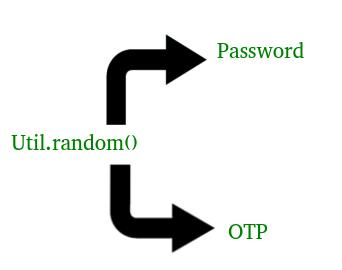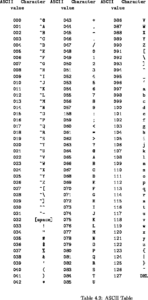Related Articles

# Generating Password and OTP in Java

• Difficulty Level : Easy
• Last Updated : 15 Oct, 2019

You may go through Generate a One Time Password or Unique Identification URL article before this for better understanding.Many a time we forget our passwords and we opt for Forget password option and within no time we get a new password at our registered email-ID or phone no. to login our account. And every time we get a different password.
Sometimes we access our bank accounts while shopping from an online store or many more ways, in order to verify our transition from the bank account, they send us OTP(One Time Password) on our registered phone no. or our email-ID, within no time.

The following code explains how to generate such Passwords and OTP within no time and what code we can use if in case we need to do so.

Method 1:

Java program explaining the generation of Password

 `// Java code to explain how to generate random``// password`` ` `// Here we are using random() method of util``// class in Java``import` `java.util.*;`` ` `public` `class` `NewClass``{``    ``public` `static` `void` `main(String[] args)``    ``{``        ``// Length of your password as I have choose``        ``// here to be 8``        ``int` `length = ``10``;``        ``System.out.println(geek_Password(length));``    ``}`` ` `    ``// This our Password generating method``    ``// We have use static here, so that we not to``    ``// make any object for it``    ``static` `char``[] geek_Password(``int` `len)``    ``{``        ``System.out.println(``"Generating password using random() : "``);``        ``System.out.print(``"Your new password is : "``);`` ` `        ``// A strong password has Cap_chars, Lower_chars,``        ``// numeric value and symbols. So we are using all of``        ``// them to generate our password``        ``String Capital_chars = ``"ABCDEFGHIJKLMNOPQRSTUVWXYZ"``;``        ``String Small_chars = ``"abcdefghijklmnopqrstuvwxyz"``;``        ``String numbers = ``"0123456789"``;``                ``String symbols = ``"!@#\$%^&*_=+-/.?<>)"``;`` ` ` ` `        ``String values = Capital_chars + Small_chars +``                        ``numbers + symbols;`` ` `        ``// Using random method``        ``Random rndm_method = ``new` `Random();`` ` `        ``char``[] password = ``new` `char``[len];`` ` `        ``for` `(``int` `i = ``0``; i < len; i++)``        ``{``            ``// Use of charAt() method : to get character value``            ``// Use of nextInt() as it is scanning the value as int``            ``password[i] =``              ``values.charAt(rndm_method.nextInt(values.length()));`` ` `        ``}``        ``return` `password;``    ``}``}`

Note : The password we are generating will change every time. As we have used random() method to generate the password.
Output :

```Generating password using random() :

Java program explaining the generation of OTP(One Time Password)

 `// Java code to explain how to generate OTP`` ` `// Here we are using random() method of util``// class in Java``import` `java.util.*;`` ` `public` `class` `NewClass``{``    ``static` `char``[] OTP(``int` `len)``    ``{``        ``System.out.println(``"Generating OTP using random() : "``);``        ``System.out.print(``"You OTP is : "``);`` ` `        ``// Using numeric values``        ``String numbers = ``"0123456789"``;`` ` `        ``// Using random method``        ``Random rndm_method = ``new` `Random();`` ` `        ``char``[] otp = ``new` `char``[len];`` ` `        ``for` `(``int` `i = ``0``; i < len; i++)``        ``{``            ``// Use of charAt() method : to get character value``            ``// Use of nextInt() as it is scanning the value as int``            ``otp[i] =``             ``numbers.charAt(rndm_method.nextInt(numbers.length()));``        ``}``        ``return` `otp;``    ``}``    ``public` `static` `void` `main(String[] args)``    ``{``        ``int` `length = ``4``;``        ``System.out.println(OTP(length));``    ``}``}`

Note :
The OTP we are generating will change every time. As we have used random() method to generate the OTP.
Output :

```Generating OTP using random() :
You OTP is : 5291```

Method 2:

Java program explaining the generation of Password

 `// Java code to explain how to generate random``// password``class` `uniquePassword``{``public` `static` `long` `Code() ``//this code returns the  unique 16 digit code ``{  ``//creating a 16 digit code using Math.random function``    ``long` `code   =(``long``)((Math.random()*``9``*Math.pow(``10``,``15``))+Math.pow(``10``,``15``));``    ``return` `code; ``//returning the code``}``    ``//method to generate the password``    ``//by converting every two digits as an ascii value of a character``public` `static` `void` `main(String args[]) ``{``long` `code=Code();``//function calling``String unique_password=``""``;``for` `(``long` `i=code;i!=``0``;i/=``100``)``//a loop extracting 2 digits from the code ``    ``{``        ``long` `digit=i%``100``;``//extracting two digits``        ``if` `(digit<=``90``)``        ``digit=digit+``32``; ``        ``//converting those two digits(ascii value) to its character value``        ``char` `ch=(``char``) digit;``        ``// adding 32 so that our least value be a valid character ``        ``unique_password=ch+unique_password;``//adding the character to the string``    ``}``    ``System.out.println(``"unique password ="``+unique_password);``    ``}``}``// Here we are using random() method of util``// class in Java`

Note : The password we are generating will change every time. As we have used random() method to generate the password.
Output :

```Generating password using Math.random() and ascii code:

#### ascii code table:Java program explaining the generation of OTP(One Time Password)

 `// Java code to explain how to generate OTP``public` `class` `GenerateOTP {``//declaring a of return type String``//which on calling provides the otp``    ``public` `static` `String generateOTP() ``    ``{  ``//int randomPin declared to store the otp``        ``//since we using Math.random() hence we have to type cast it int``        ``//because Math.random() returns decimal value``        ``int` `randomPin   =(``int``) (Math.random()*``9000``)+``1000``;``        ``String otp  = String.valueOf(randomPin);``        ``return` `otp; ``//returning value of otp``    ``}``            ``public` `static` `void` `main(String args[])``//method to call and print otp``    ``{``        ``String otpSting  =generateOTP();``//function calling``        ``System.out.println(``"OTP : "``+otpSting);``    ``}``}``// Here we are using Math.random() function.``// class in Java`

Note :
The OTP we are generating will change every time. As we have used Math.random() function to generate the OTP.
Output :

```Generating OTP using random() :
You OTP is : 5291```

This article is contributed by Mohit Gupta 🙂. If you like GeeksforGeeks and would like to contribute, you can also write an article using contribute.geeksforgeeks.org or mail your article to contribute@geeksforgeeks.org. See your article appearing on the GeeksforGeeks main page and help other Geeks.